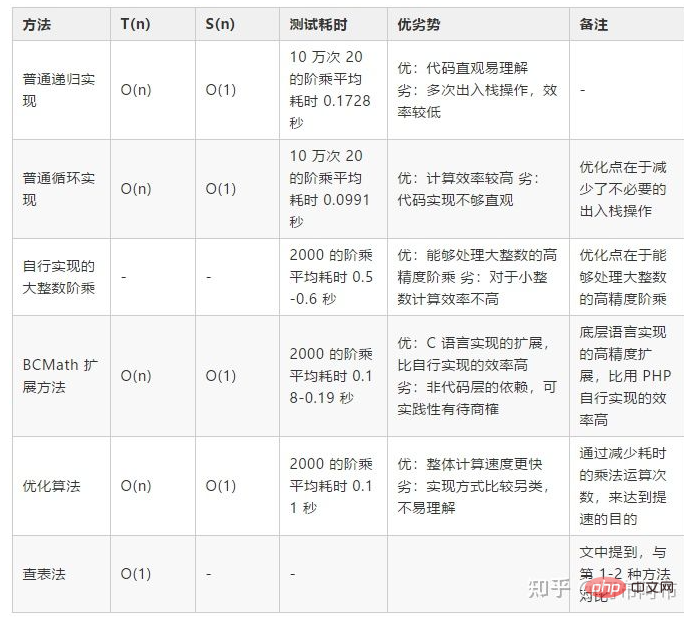# php怎么实现n的阶乘

php实现n的阶乘的方法：1、通过普通递归实现，代码如“function fact(int \$n): int{...}”；2、通过普通循环实现，代码如“while (\$num <= \$n) {\$result = \$result...}”。php怎么实现n的阶乘?

```function fact(int \$n): int
{
if (\$n == 0) {
return 1;
}
return \$n * fact(\$n - 1);
}```

```function fact(int \$n): int
{
\$result = 1;
\$num = 1;
while (\$num <= \$n) {
\$result = \$result * \$num;
\$num = \$num + 1;
}
return \$result;
}```

```function fact(int \$n): array
{
\$result = ;
\$num = 1;
while (\$num <= \$n) {
\$carry = 0;
for (\$index = 0; \$index < count(\$result); \$index++) {
\$tmp = \$result[\$index] * \$num + \$carry;
\$result[\$index] = \$tmp % 10;
\$carry = floor(\$tmp / 10);
}
while (\$carry > 0) {
\$result[] = \$carry % 10;
\$carry = floor(\$carry / 10);
}
\$num = \$num + 1;
}
return \$result;
}```

BCMath 扩展方法

BCMath 是 PHP 的一个数学扩展，用于处理字符串表示的数字（任意大小和精度）的数值计算。由于是使用 C 语言实现的扩展，计算速度会比上述自行实现的快。

```function fact(int \$n): string
{
\$result = '1';
\$num = '1';
while (\$num <= \$n) {
\$result = bcmul(\$result, \$num);
\$num = bcadd(\$num, '1');
}
return \$result;
}```

```function fact(int \$n): string
{
\$middleSquare = pow(floor(\$n / 2), 2);
\$result = \$n & 1 == 1 ? 2 * \$middleSquare * \$n : 2 * \$middleSquare;
\$result = (string)\$result;
for (\$num = 1; \$num < \$n - 2; \$num = \$num + 2) {
\$middleSquare = \$middleSquare - \$num;
\$result = bcmul(\$result, (string)\$middleSquare);
}
return \$result;
}```#### 相关文章推荐

• php小数怎么转为整数• php怎么修改错误级别• php怎么使用foreach遍历数组• php小数怎么去掉末尾0• php7和hvvm的区别是什么

### 全部评论我要评论

• 取消发布评论发送
• 1/1